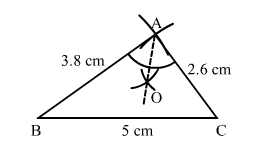# Construct a ∆ABC in which BC = 5 cm, AB = 3.8 cm and AC = 2.6 cm

Question:

Construct a ABC in which BC = 5 cm, AB = 3.8 cm and AC = 2.6 cm. Bisect the largest angle of this triangle.

Solution:Steps of construction:
1. Draw a line segment BC = 5 cm.
2. With B as the centre and a radius equal to 3.8 cm, draw an arc.
3. With C as the centre and a radius equal to 2.6 cm, draw an arc cutting the previously drawn arc at A.
4. Join AB and AC.
Thus, ABC is the required triangle.
Take the largest angle and draw the angle bisector.